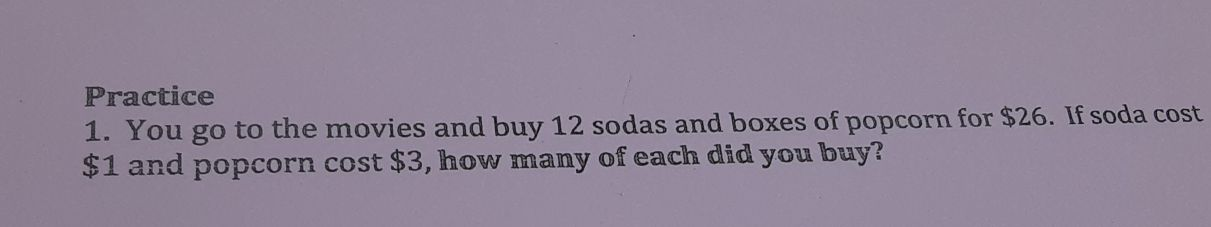### ¿Todavía tienes preguntas de matemáticas?

Pregunte a nuestros tutores expertos
Algebra
Pregunta1. You go to the movies and buy $$12$$ sodas and boxes of popcorn for $$\ 26$$ . If soda cost

$$\ 1$$ and popcorn cost $$\ 3$$ , how many of each did you buy?